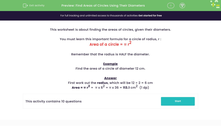# Find the Areas of Circles Using Their Diameters

In this worksheet, students will work out the areas of circles given their diameters.Key stage:  KS 3

Curriculum topic:   Geometry and Measures

Curriculum subtopic:   Solve Problems Involving Perimeters and Areas of 2D Shapes

Popular topics:   Area worksheets, Geometry worksheets

Difficulty level:#### Worksheet Overview

This activity is about finding the areas of circles, given their diameters.

You need to learn this important formula for a circle of radius, r :

Area of a circle = π r2

Remember that the radius is half the diameter.

Example

Find the area of a circle of diameter 12 cm.

First, work out the radius, which will be 12 ÷ 2 = 6 cm

Area = π r2 =  π x 62 = π x 36 = 113.1 cm2  (1 dp)

Let's have a go at some questions now.

### What is EdPlace?

We're your National Curriculum aligned online education content provider helping each child succeed in English, maths and science from year 1 to GCSE. With an EdPlace account you’ll be able to track and measure progress, helping each child achieve their best. We build confidence and attainment by personalising each child’s learning at a level that suits them.

Get started